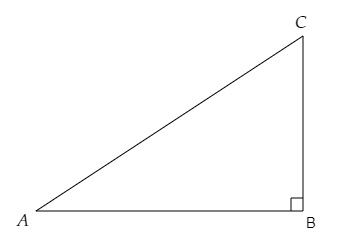# In each of the following, one of the six trigonometric ratios is given. Find the values of the other trigonometric ratios.$\sin \theta=\frac{11}{15}$

Given:

$\sin \theta=\frac{11}{15}$

To do:

We have to find the values of the other trigonometric ratios.

Solution:

We know that,In a right-angled triangle $ABC$ with right angle at $B$,

By Pythagoras theorem,

$AC^2=AB^2+BC^2$

By trigonometric ratios definitions,

$sin\ A=\frac{Opposite}{Hypotenuse}=\frac{BC}{AC}$

$cos\ A=\frac{Adjacent}{Hypotenuse}=\frac{AB}{AC}$

$tan\ A=\frac{Opposite}{Adjacent}=\frac{BC}{AB}$

$cosec\ A=\frac{Hypotenuse}{Opposite}=\frac{AC}{BC}$

$sec\ A=\frac{Hypotenuse}{Adjacent}=\frac{AC}{AB}$

$cot\ A=\frac{Adjacent}{Opposite}=\frac{AB}{BC}$

Here,

Let $sin\ \theta=\frac{BC}{AC}=\frac{11}{15}$

$AC^2=AB^2+BC^2$

$\Rightarrow (15)^2=(AB)^2+(11)^2$

$\Rightarrow AB^2=225-121$

$\Rightarrow AB=\sqrt{104}=\sqrt{4\times26}=2\sqrt{26}$

Therefore,

$cos\ \theta=\frac{AB}{AC}=\frac{2\sqrt{26}}{15}$

$tan\ \theta=\frac{BC}{AB}=\frac{11}{2\sqrt{26}}$

$cosec\ \theta=\frac{AC}{BC}=\frac{15}{11}$

$sec\ \theta=\frac{AC}{AB}=\frac{15}{2\sqrt{26}}$

$cot\ \theta=\frac{AB}{BC}=\frac{2\sqrt{26}}{11}$